# Differential expression analysis

The goal of differential expression analysis is to perform statistical analysis to try and discover changes in expression levels of defined features (genes, transcripts, exons) between experimental groups with replicated samples.

Most of the popular tools for differential expression analysis are available as R / Bioconductor packages.
Bioconductor is an R project and repository that provides a set of packages and methods for omics data analysis.

The best performing tools for differential expression analysis tend to be:

See Schurch et al, 2015; arXiv:1505.02017 and the Biostars thread about the main differences between the methods.

In this tutorial, we will give you an overview of the DESeq2 pipeline to find differentially expressed genes between two conditions.

## DESeq2

DESeq2 is an R/Bioconductor implemented method to detect differentially expressed features.
It tests for differential expression using negative binomial generalized linear models.
DESeq2 (as edgeR) is based on the hypothesis that most genes are not differentially expressed.

This DESeq2 tutorial is inspired by the RNA-seq workflow developped by the authors of the tool, and by the differential gene expression course from the Harvard Chan Bioinformatics Core.

DESeq2 takes as an input raw counts (i.e. non normalized counts): the DESeq2 model internally corrects for library size, so giving as an input normalized count would be incorrect.

### DESeq2 steps:

• Modeling raw counts for each gene:
• Estimate size factors (accounts for differences in library size).
• Estimate dispersions.
• GLM (Generalized Linear Model) fit for each gene.
• Testing for differential expression (Wald test).
DESeq2 uses the median of ratio method for normalization: briefly, the counts are divided by sample-specific size factors.
Geometric mean is calculated for each gene across all samples. The counts for a gene in each sample is then divided by this mean. The median of these ratios in a sample is the size factor for that sample.
For additional information regarding the tool and the algorithm, please refer to the paper and the user-friendly package vignette.

### Tutorial on basic DESeq2 usage for differential analysis of gene expression

• In this tutorial, we will use the counts calculated from the mapping on all chromosomes (we practiced so far QC and mapping for data of only one chromosome but here we consider all chromosomes), for the 10 samples previously selected from GEO:
 GEO ID SRA ID Sample name Differentiation Condition GSM2031982 SRR3091420 5p4_25c undiff WT GSM2031983 SRR3091421 5p4_27c undiff WT GSM2031984 SRR3091422 5p4_28c diff 5 days WT GSM2031985 SRR3091423 5p4_29c diff 5 days WT GSM2031986 SRR3091424 5p4_30c diff 5 days WT GSM2031987 SRR3091425 5p4_31cfoxc1 undiff KO GSM2031988 SRR3091426 5p4_32cfoxc1 undiff KO GSM2031989 SRR3091427 5p4_33cfoxc1 undiff KO GSM2031990 SRR3091428 5p4_34cfoxc1 diff 5 days KO GSM2031991 SRR3091429 5p4_35cfoxc1 diff 5 days KO

The FOXC1 protein plays a critical role in early development, particularly in the formation of structures in the front part of the eye (the anterior segment). These structures include the colored part of the eye (the iris), the lens of the eye, and the clear front covering of the eye (the cornea). Studies suggest that the FOXC1 protein may also have functions in the adult eye, such as helping cells respond to oxidative stress. Oxidative stress occurs when unstable molecules called free radicals accumulate to levels that can damage or kill cells. The FOXC1 protein is also involved in the normal development of other parts of the body, including the heart, kidneys, and brain. Source.

Get the count data for the full data set, output of both STAR and Salmon:

``````# Go to the differential expression directory
cd ~/rnaseq_course/differential_expression

# Get the folder containing all the data
wget https://public-docs.crg.es/biocore/projects/training/PHINDaccess2020/full_data_counts.tar.gz

# Gunzip
tar -zxvf full_data_counts.tar.gz

# Remove full_data.tar.gz once extraction is completed
rm full_data_counts.tar.gz
``````

### Raw count matrices

DESeq2 takes as an input raw (non normalized) counts, in various forms:

• A matrix for all sample
• One file per sample (our option for STAR)
• A txi object (our option for Salmon)

#### Prepare data from STAR

We need to create one file per sample, each file containing the raw counts of all genes:

File SRR3091420_1_chr6_counts.txt:

 ENSG00000260370.1 0 ENSG00000237297.1 10 ENSG00000261456.5 210

File SRR3091421_1_chr6_counts.txt:

 ENSG00000260370.1 0 ENSG00000237297.1 8 ENSG00000261456.5 320

and so on…
Remember that the STAR count file contains 4 columns depending on the library preparation protocol!

Exercise

• Prepare the 10 files needed for our analysis, from the STAR output, and save them in the counts_selected directory: knowing that our libraries are unstranded, which column will you pick?
• Create the sub-directory counts_STAR_selected inside the deseq2 directory:
``````mkdir ~/rnaseq_course/differential_expression/counts_STAR_selected
``````
• Loop around the 10 ReadsPerGene.out.tab files and extract the gene ID (1rst column) and the correct counts (2nd column).
``````cd ~/rnaseq_course/differential_expression

do echo \$i
# retrieve the first (gene name) and second column (raw reads for unstranded protocol)
cut -f1,2 \$i | grep -v "_" > counts_STAR_selected/\$(basename \$i ReadsPerGene.out.tab)_counts.txt
done
``````

#### Prepare transcript-to-gene annotation file

Prepare the annotation file needed to import the Salmon counts: a two-column data frame linking transcript id (column 1) to gene id (column 2).
We will add the gene symbol in column 3, for a more comprehensive annotation.
Process from the GTF file:

``````cd ~/rnaseq_course/differential_expression

wget ftp://ftp.ebi.ac.uk/pub/databases/gencode/Gencode_human/release_33/gencode.v33.annotation.gtf.gz

# first column is the transcript ID, second column is the gene ID, third column is the gene symbol
zcat gencode.v33.annotation.gtf.gz | awk -F "\t" 'BEGIN{OFS="\t"}{if(\$3=="transcript"){split(\$9, a, "\""); print a,a,a}}' > tx2gene.gencode.v33.csv

``````

#### Sample sheet

Additionally, DESeq2 needs a sample sheet that describes the samples characteristics: treatment, knock-out / wild type, replicates, time points, etc. in the form:

SampleName FileName Differentiation Condition
5p4_25c SRR3091420_1_counts.txt undiff WT
5p4_27c SRR3091421_1_counts.txt undiff WT
5p4_28c SRR3091422_1_counts.txt diff5days WT
5p4_29c SRR3091423_1_counts.txt diff5days WT
5p4_30c SRR3091424_1_counts.txt diff5days WT
5p4_31cfoxc1 SRR3091425_1_counts.txt undiff KO
5p4_32cfoxc1 SRR3091426_1_counts.txt undiff KO
5p4_33cfoxc1 SRR3091427_1_counts.txt undiff KO
5p4_34cfoxc1 SRR3091428_1_counts.txt diff5days KO
5p4_35cfoxc1 SRR3091429_1_counts.txt diff5days KO

The first column is the sample name, the second column the file name of the count file generated by STAR (after selection of the appropriate column as we just did), and the remaining columns are description of the samples, some of which will be used in the statistical design.

The design indicates how to model the samples: in the model we need to specify what we want to measure and what we want to control.

Exercise

• Prepare this file (tab-separated columns) in a text editor: save it as sample_sheet_foxc1.txt in the differential_analysis directory: you can do it “manually” using a text editor, or you can try using the command line.

Note that the same sample sheet will be used for both the STAR and the Salmon DESeq2 analysis. (with a slight modification that we will see later on)

BACK UP

``````wget https://public-docs.crg.es/biocore/projects/training/PHINDaccess2020/sample_sheet_foxc1.txt
``````

#### Analysis

The analysis is done in R !

Start R Studio.

Note that in the R code boxes below # is followed by comments, i.e. words not interpreted by R, as in Linux.

• Go to the differential_expression working directory and load the DESeq2 package (loading a package in R allows to use specific sets of functions developped as part of this package).
``````# setwd = set working directory; equivalent to the Linux "cd".
# the R equivalent to the Linux pwd is getwd() = get working directory.
setwd("~/rnaseq_course/differential_expression")

# load package DESeq2 (all functions)
library(DESeq2)
``````
##### Import STAR counts
• Read in the sample table that we prepared:
``````# read in the sample sheet
# header = TRUE: the first row is the "header", i.e. it contains the column names.
# sep = "\t": the columns/fields are separated with tabs.

# add the SRA codes as row names (it is needed for some of the DESeq functions)
rownames(sampletable) <- gsub("_counts.txt", "", sampletable\$FileName)

# display the first 6 rows

# check the number of rows and the number of columns
nrow(sampletable) # if this is not 10, please raise your hand !
ncol(sampletable) # if this is not 4, also raise your hand !
``````
• Load the count data from STAR into an DESeq object:
• sampleTable: the sample sheet / metadata we created
• directory: path to the directory where the counts are stored (one file per sample)
• design: design formula describing which variables will be used to model the data. Here we want to compare the experimental groups in “Condition”.
``````# Import STAR counts
se_star <- DESeqDataSetFromHTSeqCount(sampleTable = sampletable,
directory = "counts_STAR_selected",
design = ~ Condition)
``````

NOTE: the design formula is used to estimate the dispersions and to estimate the log2 fold changes of the model!

##### Import Salmon counts
• Load the count data from SALMON into an DESeq object:
``````# Go to the deseq2 directory
setwd("~/rnaseq_course/differential_expression")

# Load the tximport package that we use to import Salmon counts
library(tximport)

# List the quantification files from Salmon: one quant.sf file per sample
# dir is list all files in "~/rnaseq_course/differential_expression/counts_salmon" and in any directories inside, that have the pattern "quant.sf". full.names = TRUE means that we want to keep the whole paths
files <- dir("~/rnaseq_course/differential_expression/counts_salmon", recursive=TRUE, pattern="quant.sf", full.names=TRUE)
# files is a vector of file paths. we will name each element of this vector with a simplified corresponding sample name
names(files) <- gsub("_quant.sf", "", dir("~/rnaseq_course/differential_expression/counts_salmon"))

# Read in the two-column data.frame linking transcript id (column 1) to gene id (column 2)
sep="\t",

# tximport can import data from Salmon, Kallisto, Sailfish, RSEM, Stringtie
# here we summarize the transcript-level counts to gene-level counts
txi <- tximport(files,
type = "salmon",
tx2gene = transcripts2genes)

# check the names of the "slots" of the txi object
names(txi)

# display the first rows of the counts per gene information

# Create a DESeq2 object based on Salmon per-gene counts
se_salmon <- DESeqDataSetFromTximport(txi,
colData = sampletable,
design = ~ Condition)

``````
• From that step on, you can proceed the same way with se_star and se_salmon ! The only thing that differs slightly is the annotation (remember that for STAR we used ENSEMBL annotation while we used GENCODE annotation for Salmon)
• We will focus the rest of the analysis on the se_star.
##### Filtering out lowly expressed genes

From DESeq2 vignette: While it is not necessary to pre-filter low count genes before running the DESeq2 functions, there are two reasons which make pre-filtering useful: by removing rows in which there are very few reads, we reduce the memory size of the dds data object, and we increase the speed of the transformation and testing functions within DESeq2.

Let’s filter:

``````# Number of genes before filtering:
nrow(se_star)

# Filter
se_star <- se_star[rowSums(counts(se_star)) > 10, ]

# Number of genes left after low-count filtering:
nrow(se_star)
``````
##### Prepare annotation

The biomaRt package is used for adding a more detailed annotation to our data sets.
Additionally to the ENSEMBL gene IDs we want (for example):

• The external gene name (symbol)
• A more thorough gene description
• Chromosome and coordinates
``````# load library
library(biomaRt)

# list ENSEMBL archives
listEnsemblArchives()
# We used version 88 of ENSEMBL, which corresponds to URL http://mar2017.archive.ensembl.org

# we can load the corresponding database
mart <- useMart(biomart="ENSEMBL_MART_ENSEMBL", host="mar2017.archive.ensembl.org", path="/biomart/martservice", dataset="hsapiens_gene_ensembl")

# "filters" correspond to the input WE want to retrieve more annotation for
# a list of available filters can be obtained with listFilters(mart)

# let's see what is available in terms of ensembl ID
grep("ensembl", listFilters(mart)[,1], value=TRUE)
# ensembl_gene_id is what we want!

# "attributes" correspond to the kind of annotation you want to retrieve
# a list of available attributes can be obtained with listAttributes(mart)
# you can browse and decide what is interesting for you. For this exercise, we will use 'ensembl_gene_id', 'chromosome_name', 'start_position', 'end_position', 'description', 'external_gene_name'

# list of ENSEMBL IDs we want to annotate
gene_ids <- rownames(se_star)
# 18025 IDs

# annotate!
annot <- getBM(attributes=c('ensembl_gene_id', 'chromosome_name', 'start_position', 'end_position', 'description', 'external_gene_name'), filters ='ensembl_gene_id', values = gene_ids, mart = mart)

dim(annot)
# 18025 rows

``````
##### Fit statistical model

All steps are wrapped up in the DESeq function:

• estimating size factors
• estimating dispersions
• gene-wise dispersion estimates
• mean-dispersion relationship
• final dispersion estimates
• fitting model and testing
``````se_star2 <- DESeq(se_star)
``````
• Save the normalized counts for further usage (functional analysis, tomorrow…)
``````# compute normalized counts (log2 transformed); + 1 is a count added to avoid errors during the log2 transformation: log2(0) gives an infinite number, but log2(1) is 0.
# normalized = TRUE: divide the counts by the size factors calculated by the DESeq function
norm_counts <- log2(counts(se_star2, normalized = TRUE)+1)

norm_counts_symbols <- merge(data.frame(ID=rownames(norm_counts), norm_counts, check.names=FALSE), annot, by.x="ID", by.y="ensembl_gene_id", all=F)

# write normalized counts to text file
write.table(norm_counts_symbols, "normalized_counts_log2_star.txt", quote=F, col.names=T, row.names=F, sep="\t")
``````

Exercise

• What are the normalized counts corresponding to genes “ENSG00000169813” and “ENSG00000182898” ?
• Calculate the average and the standard deviation of these genes normalized counts. How do they differ ? What can you tell about them ?

#### Visualization

• Transform raw counts to be able to visualize the data

DESeq2 developpers advice to use transformed counts, rather than normalized counts, for anything involving a distance (e.g. visualization).
They offer to choose between two transformation methods, both of which stabilize the variance across the mean:

• rlog (Regularized log)
• VST (Variance Stabilizing Transformation)
Both options produce log2 scale data which has been normalized by the DESeq2 method with respect to library size.
From this tutorial:
The VST is much faster to compute and is less sensitive to high count outliers than the rlog. The rlog tends to work well on small datasets (n < 30), potentially outperforming the VST when there is a wide range of sequencing depth across samples (an order of magnitude difference). We therefore recommend the VST for medium-to-large datasets (n > 30).*

We have a 10 sample dataset: Let’s use the rlog transformation.
As a homework, you can try and use the VST transformation (function vst).

``````# Try with the rlog transformation
se_rlog <- rlog(se_star2)

# You can also try the same with the rlog transformation as a homework, running: rld <- rlog(se_star2)
``````
• Samples correlation

Calculate the sample-to-sample distances:

``````# load libraries pheatmap to create the heatmap plot
library(pheatmap)

# calculate between-sample distance matrix
sampleDistMatrix <- as.matrix(dist(t(assay(se_rlog))))

# prepare a "metadata" object to add a colored bar with the differentiation and condition information

# create figure in PNG format
png("sample_distance_heatmap_star.png")
# close PNG file after writing figure in it
dev.off()
``````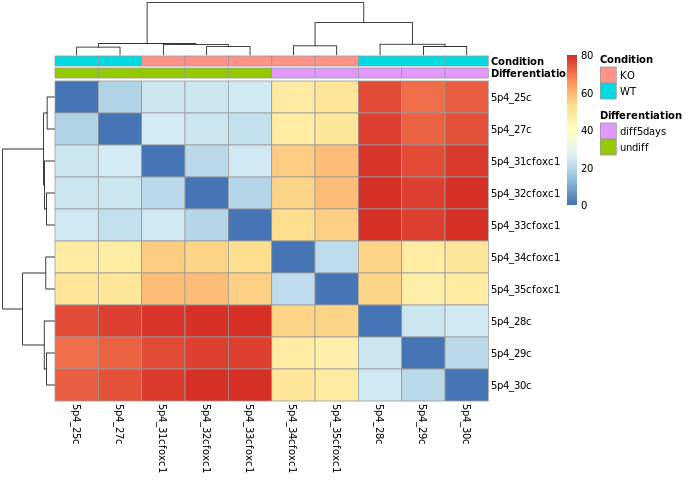Do samples cluster how you would expect ?

GET FILES WE COULD NOT CREATE YESTERDAY

``````wget https://public-docs.crg.es/biocore/projects/training/PHINDaccess2020/normalized_counts_log2_star.txt
wget https://public-docs.crg.es/biocore/projects/training/PHINDaccess2020/annotation_ens88.txt
``````

``````# biomaRt annotations
# normalized counts, annotated
``````
• Principal Component Analysis (PCA)

Reduction of dimensionality to be able to retrieve main differences / underlying variance between samples.
It is used to bring out strong patterns from complex biological datasets.
More on PCA in this post

``````png("PCA_star.png")
plotPCA(object = se_rlog,
intgroup = c("Condition", "Differentiation"))
dev.off()
``````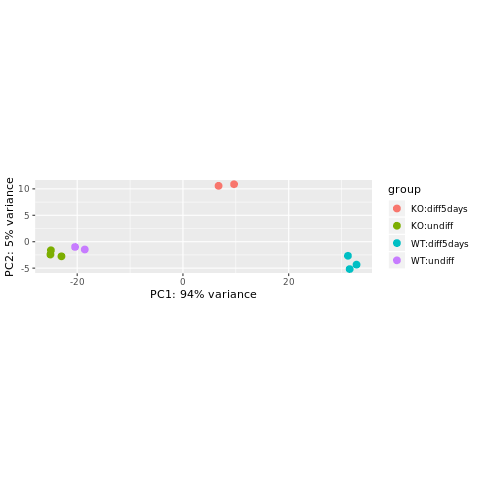The horizontal axis (PC1 = Principal Component 1) represents the highest variation between the samples. Differences along PC1 are more important than differences along PC2.

We can also plot the normalized counts of a gene per sample / experimental group:

``````# FOXC1 is ENSG00000054598
plotCounts(se_star2, gene="ENSG00000054598", intgroup="Condition")
``````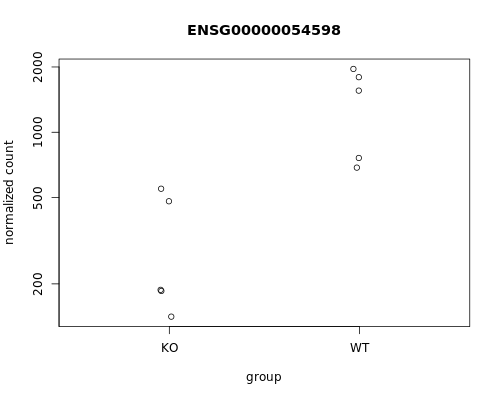Let’s produce a more comprehensive plot: we can add the sample names and the differentiation status.
To do so, we can use the ggplot2 package.

``````library(ggplot2)
library(reshape2)

# Retrieve the normalized counts per sample for FOXC1 / ENSG00000054598
tmp <- norm_counts[rownames(norm_counts)=="ENSG00000054598",]

# convert to "long" format
mygenelong <- melt(tmp)

# sample name
mygenelong\$name <- rownames(mygenelong)

# sample Condition and Differentiation: merge with sample table
mygenelong <- merge(mygenelong, sampletable, by.x="name", by.y="SampleName", all=F)

# Dot plot
pdot <- ggplot(data=mygenelong, mapping=aes(x=Condition, y=value, col=Differentiation, shape=Condition, label=name)) +
geom_point() +
geom_text(nudge_x=0.2) +
xlab(label="Experimental group") +
ylab(label="Normalized expression (log2)") +
theme_bw()
``````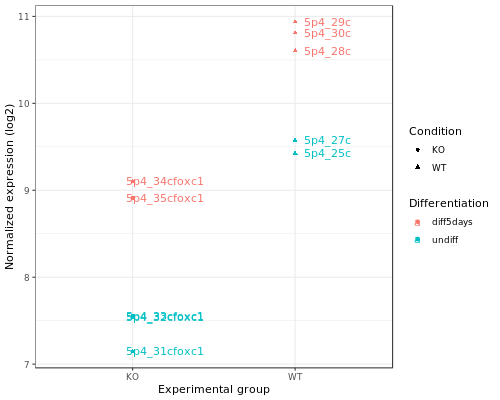##### Differential expression analysis
``````# check results names: depends on what was modeled. Here it was the "Condition"
resultsNames(se_star2)

# extract results for WT vs KO
# contrast: the column from the metadata that is used for the grouping of the samples (Condition), then WT is compared to the KO -> results will be as "WT vs KO"
de <- results(object = se_star2,
name="Condition_WT_vs_KO")
# This is equivalent to:
de <- results(object = se_star2, contrast=c("Condition", "WT", "KO"))

# If you want the results to be expressed as "KO vs WT", you can run:
# de <- results(object = se_star2, contrast=c("Condition", "KO", "WT"))

# check first rows

# add more annotation to "de"
de_symbols <- merge(data.frame(ID=rownames(de), de, check.names=FALSE), annot, by.x="ID", by.y="ensembl_gene_id", all=F)

# write differential expression analysis result to a text file
write.table(de_symbols, "deseq2_results.txt", quote=F, col.names=T, row.names=F, sep="\t")
``````

#### DESeq2 output

• log2 fold change:
A positive fold change indicates an increase of expression while a negative fold change indicates a decrease in expression for a given comparison.
This value is reported in a logarithmic scale (base 2): for example, a log2 fold change of 1.5 in the “WT vs KO comparison” means that the expression of that gene is increased, in the WT relative to the KO, by a multiplicative factor of 2^1.5 ≈ 2.82.
• pvalue: Wald test p-value: Indicates whether the gene analysed is likely to be differentially expressed in that comparison. The lower the more significant.
• padj: Bonferroni-Hochberg adjusted p-values (FDR): the lower the more significant. More robust that the regular p-value because it controls for the occurrence of false positives.
• baseMean: Mean of normalized counts for all samples.
• lfcSE: Standard error of the log2FoldChange.
• stat: Wald statistic: the log2FoldChange divided by its standard error.

Note on p-values set to NA: some values in the results table can be set to NA for one of the following reasons: (from Analyzing RNA-seq data with DESeq2 by M. Love et al., 2017

• If within a row, all samples have zero counts, the baseMean column will be zero, and the log2 fold change estimates, p value and adjusted p value will all be set to NA.
• If a row contains a sample with an extreme count outlier then the p value and adjusted p value will be set to NA. These outlier counts are detected by Cook’s distance. If there are very many outliers (e.g. many hundreds or thousands) reported by summary(res), one might consider further exploration to see if a single sample or a few samples should be removed due to low quality.
• If a row is filtered by automatic independent filtering, for having a low mean normalized count, then only the adjusted p value will be set to NA. This independent filtering can be customized or turned off in the DESeq2 function results(dds, independentFiltering=FALSE).

#### Gene selection

• padj (p-value corrected for multiple testing)
• log2FC (log2 Fold Change)

the log2FoldChange gives a quantitative information about the expression changes, but does not give an information on the within-group variability, hence the reliability of the information:

In the picture below, fold changes for gene A and for gene B between groups t25 and t0 (from another data set) are the same, however the variability between the replicated samples in gene B is higher, so the result for gene A will be more reliable (i.e. the p-value will be smaller).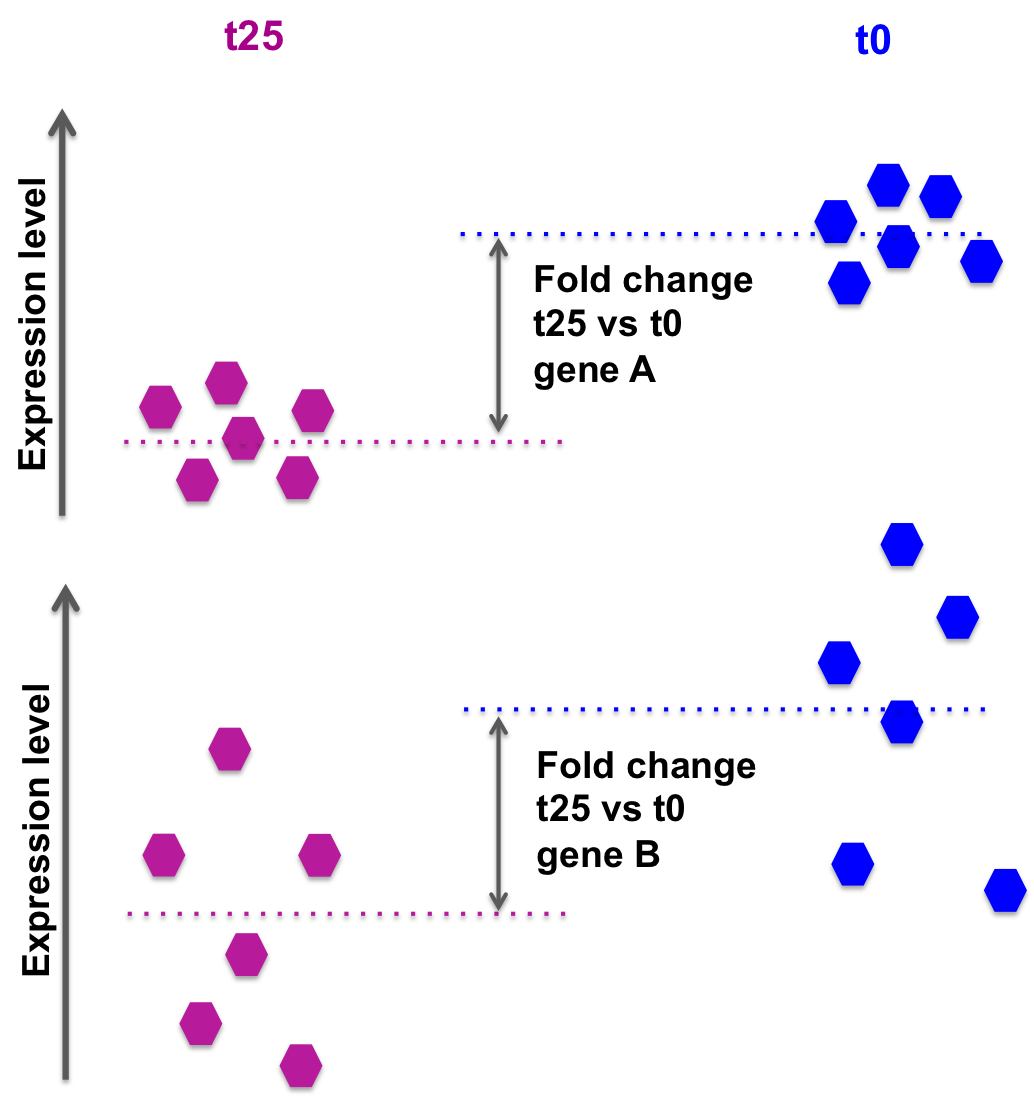DESeq2 also takes into account the library size, sufficient coverage of a gene, …

We need to take into account the p-value or, better the adjusted p-value (padj).

Setting a p-value threshold of 0.05 means that there is a 5% chance that the observed result is a false positive.
For thousands of simultaneous tests (as in RNA-seq, there are thousands of genes tested at the same time), 5% can result in a large number of false positives.

The Benjamini-Hochberg procedure controls the False Discovery Rate (FDR) (it is one of many methods to adjust p-values for multiple tetsing).
A FDR adjusted p-value of 0.05 implies that 5% of significant tests according to the “raw” p-value will result in false positives.

• Selection of differentially expressed genes between WT and KO based on padj < 0.05.
``````# how many genes are differentially expressed, taking into account "padj < 0.05"?
# contains NAs... Filter them out
# 84 genes

# save results in file for further usage
write.table(de_select, "deseq2_selection_padj005.txt", quote=F, col.names=T, row.names=F, sep="\t")
``````
• Selection of differentially expressed genes between WT and KO based on padj < 0.05 AND log2FC > 0.5 or log2FC < -0.5 (However, note that selecting by log2FoldChange is not required if the selection is done using the padj).
``````# how many genes are differentially expressed, taking into account "padj < 0.05" and log2FoldChange < -0.5 or > 0.5?
# contains NAs... Filter them out
# 82 genes
``````

Exercise 1

• Is FOXC1 differentially expressed? What are the corresponding adjusted-value and log2FoldChanges?
• How many genes are found differentially expressed if you change the log2FoldChange threshold to 0.8 / -0.8 and the padj threshold to 0.01 ?

Exercise 2

• Repeat the analysis comparing WT vs KO for the undifferentiated samples only!
• Steps are:
• Modify the “sampletable” so that it contains only samples corresponding to “undiff” Differentiation state.
SampleName FileName Differentiation Condition
5p4_25c SRR3091420_1_counts.txt undiff WT
5p4_27c SRR3091421_1_counts.txt undiff WT
5p4_31cfoxc1 SRR3091425_1_counts.txt undiff KO
5p4_32cfoxc1 SRR3091426_1_counts.txt undiff KO
5p4_33cfoxc1 SRR3091427_1_counts.txt undiff KO
• Filter out low counts (keep high counts)
• Fit statistical model DESeq()
• rlog-transform counts rlog()
• Plot PCA and sample-to-sample distances heatmap
• Check differential expression resultsNames()
• How many genes are differentially expressed, when considering padj < 0.05? DON’T FORGET TO WRITE FILES DOWN AT EACH STEP!!

STEP BY STEP CORRECTION

``````## DESeq2 analysis

library(DESeq2)

# Create sample sheet

sampletable <- data.frame(SampleName=c("5p4_25c", "5p4_27c", "5p4_28c", "5p4_29c", "5p4_30c", "5p4_31cfoxc1", "5p4_32cfoxc1", "5p4_33cfoxc1", "5p4_34cfoxc1", "5p4_35cfoxc1"),
FileName=c("SRR3091420_1_counts.txt", "SRR3091421_1_counts.txt", "SRR3091422_1_counts.txt", "SRR3091423_1_counts.txt", "SRR3091424_1_counts.txt", "SRR3091425_1_counts.txt", "SRR3091426_1_counts.txt", "SRR3091427_1_counts.txt", "SRR3091428_1_counts.txt", "SRR3091429_1_counts.txt"),
Differentiation=c(rep("undiff", 2), rep("diff5days", 3), rep("undiff", 3), rep("diff5days", 2)),
Condition=c(rep("WT", 5), rep("KO", 5)))
rownames(sampletable) <- gsub("_counts.txt", "", sampletable\$FileName)

# Modify sample sheet to keep only "undiff" samples

sampletable2 <- sampletable[sampletable\$Differentiation=="undiff",]

# Import STAR counts
se_star <- DESeqDataSetFromHTSeqCount(sampleTable = sampletable2,
directory = "counts_STAR_selected",
design = ~ Condition)

# Filter out lowly expressed genes
# i.e. (keep genes for which sums of raw counts across experimental samples is > 10)

se_star <- se_star[rowSums(counts(se_star)) > 10, ]

# Annotate
gene_ids <- rownames(se_star)

library(biomaRt)

mart <- useMart(biomart="ENSEMBL_MART_ENSEMBL", host="mar2017.archive.ensembl.org", path="/biomart/martservice", dataset="hsapiens_gene_ensembl")

annot <- getBM(attributes=c('ensembl_gene_id', 'chromosome_name', 'start_position', 'end_position', 'description', 'external_gene_name'), filters ='ensembl_gene_id', values = gene_ids, mart = mart)

# Fit statistical model

se_star2 <- DESeq(se_star)

# Compute normalized counts
norm_counts <- log2(counts(se_star2, normalized = TRUE)+1)

# add annotation to count table
norm_counts_symbols <- merge(data.frame(ID=rownames(norm_counts), norm_counts, check.names=FALSE), annot, by.x="ID", by.y="ensembl_gene_id", all=F)

# write normalized counts to text file
write.table(norm_counts_symbols, "normalized_counts_log2_star_undiff.txt", quote=F, col.names=T, row.names=F, sep="\t")

# Transform counts for visualization
se_rlog <- rlog(se_star2)

# Build heatmap
# load libraries pheatmap to create the heatmap plot
library(pheatmap)

# calculate between-sample distance matrix
sampleDistMatrix <- as.matrix(dist(t(assay(se_rlog))))

# prepare a "metadata" object to add a colored bar with the differentiation and condition information

# create figure in PNG format
png("sample_distance_heatmap_star_undiff.png")
# close PNG file after writing figure in it
dev.off()

# Principal component analysis
png("PCA_star_undiff.png")
plotPCA(object = se_rlog,
intgroup = c("Condition", "Differentiation"))
dev.off()

# Differential expression analysis
de <- results(object = se_star2,
name="Condition_WT_vs_KO")
de_symbols <- merge(data.frame(ID=rownames(de), de, check.names=FALSE), annot, by.x="ID", by.y="ensembl_gene_id", all=F)

# write differential expression analysis result to a text file
write.table(de_symbols, "deseq2_results_undiff.txt", quote=F, col.names=T, row.names=F, sep="\t")

# Select genes for which padj < 0.05

# save results in file for further usage
write.table(de_select, "deseq2_selection_padj005_undiff.txt", quote=F, col.names=T, row.names=F, sep="\t")
``````

Exercise 3

##### Control for “Differentiation”

While in Exercise 2 we tested WT vs KO on undifferentiated samples only, we can also use a more complex design formula. If we specify:

``````~ Differentiation + Condition
``````

it means that we want to test for the effect of the FOXC1 knock out, while controlling for the effect of differentiation.
In a way, we “discard” the expected changes due to differentiation to focus on the changes specifically driven by the KO.

• Repeat the first analysis, changing the design ~ Condition to ~ Differentiation + Condition.
• How many genes are now found differentially expressed, when filtering for padj < 0.05?

Homework

Do the same using the Salmon counts (object se_salmon): how many genes are found differentially expressed when using the Salmon counts ?
How do results overlap between STAR and Salmon ?